## How to convert letter to number or vice versa in Excel?

If you have a list of letters of an alphabet, and now you want to convert these letters to their relative numbers, such as A=1, B=2, C=3… And in other cases, you need to reverse this option to change the numbers to their associated letters. Have you ever tried to solve this task in Excel worksheet?

#### Convert letter to number with formula

To convert the letters to relative numbers, you can use the following formula:

1. In a blank cell besides your data, for example, cell D2, please enter below formula, and press Enter key.

=COLUMN(INDIRECT(B3&1))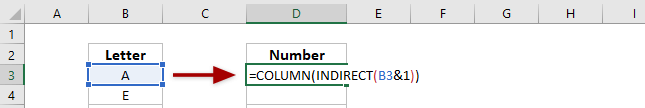2. Then drag the fill handle over to the range that you want to apply this formula, and the letters have been converted to their relative numbers as below screenshot shown: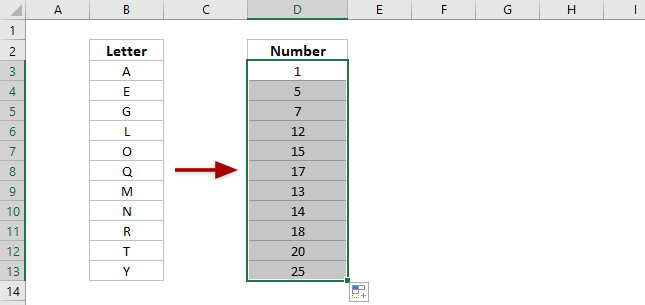### Easily convert multiple numbers to currency words in bulk in Excel

It’s easy to manually convert the currency \$357.6 to the currency word of three hundred fifty-seven dollars and sixty cents. But how about converting hundreds of numbers to currency words. Use the amazing Numbers to Currency Words feature of Kutools of Excel, and batch convert numbers to currency words easily in Excel.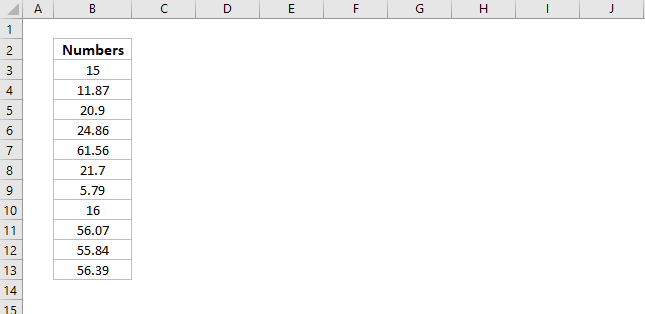Kutools for Excel - Includes more than 300 handy tools for Excel. Full feature free trial 30-day, no credit card required! Get It Now

#### Convert number to letter with formula

If you want to convert the integer numbers which are in the range of 1 to 26 to the corresponding letters, the following formula can do you a favor.

1. In a blank cell besides your data, for example, cell D3, please enter below formula, and press the Enter key.

=MID("ABCDEFGHIJKLMNOPQRSTUVWXYZ",B3,1)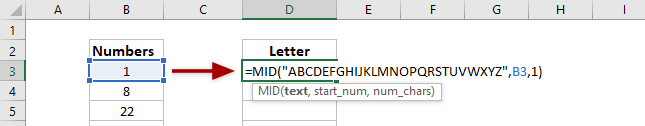2. Then drag the fill handle over to the range that you want to contain this formula, and the numbers have been converted to their relative letters as you need. See screenshot: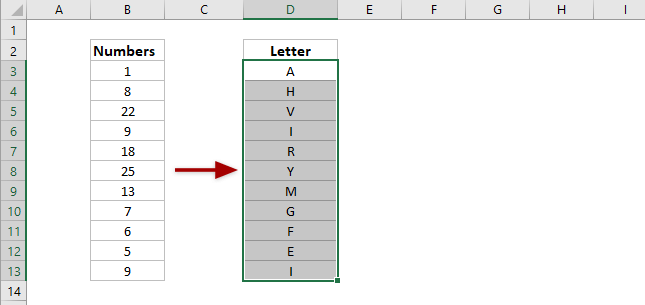Tip:
(1) If the number is greater than 26, the formula will return empty;
(2) If the number is less than 1, the formula will return #VALUE! error value;
(3) If the number is a decimal number, the formula will trim number to a whole number and then calculate.

#### Convert number to currency text with an amazing tool

If have installed Kutools for Excel, you can use its Numbers to Currency Words feature to convert all selected numbers to currency words in bulk in Excel.

Kutools for Excel- Includes more than 300 handy tools for Excel. Full feature free trial 60-day, no credit card required! Get It Now

1. Select the numbers you will convert to currency word, and click Kutools > Content > Numbers to Currency Words.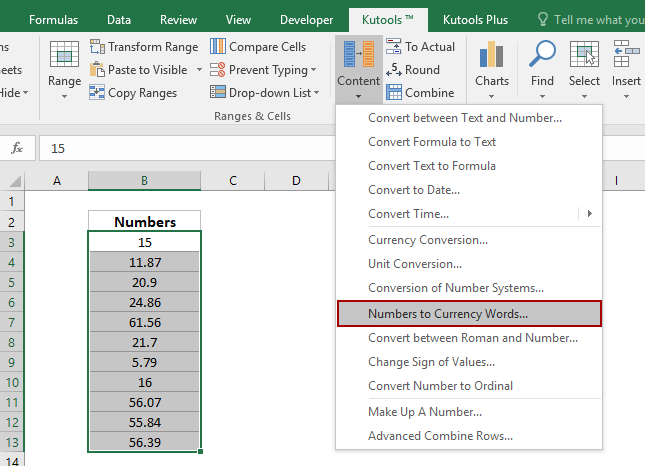2. In the Numbers to Currency Words dialog, please check English or Chinese option as you need, and click the Ok button.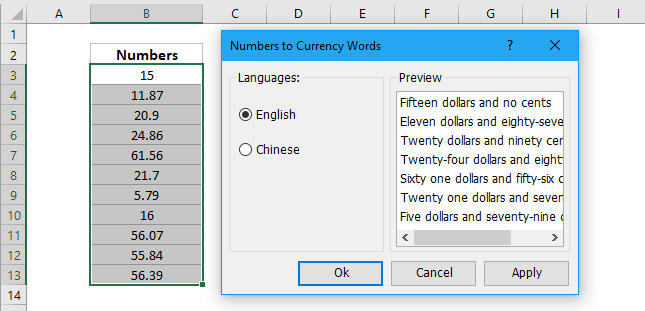Now you will see all selected numbers are converted to currency words in bulk. See screenshot: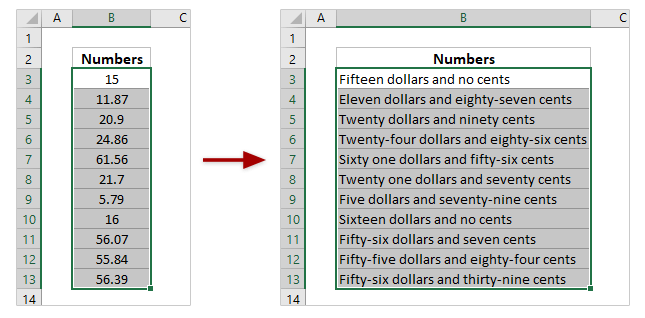### Best Office Productivity Tools

Supercharge Your Spreadsheets： Experience Efficiency Like Never Before with Kutools for Excel

 Popular Features: Find/Highlight/Identify Duplicates   |  Delete Blank Rows   |  Combine Columns or Cells without Losing Data   |   Round without Formula ... Super Lookup: Multiple Criteria VLookup  |   Multiple Value VLookup  |   VLookup Across Multiple Sheets   |   Fuzzy Lookup .... Advanced Drop-down List: Quickly Create Drop Down List   |  Dependent Drop Down List   |  Multi-select Drop Down List .... Column Manager: Add a Specific Number of Columns   |   Move Columns   |   Unhide Columns   |   Compare Columns to Select Same & Different Cells ... Featured Features: Grid Focus   |  Design View   |   Big Formula Bar   |  Workbook & Sheet Manager   |  Resource Library (Auto Text)   |  Date Picker   |  Combine Worksheets   |  Encrypt/Decrypt Cells   |  Send Emails by List   |  Super Filter   |   Special Filter (filter bold/italic/strikethrough...) ... Top 15 Toolset:  12 Text Tools (Add Text, Remove Characters, ...)   |   50+ Chart Types (Gantt Chart, ...)   |   40+ Practical Formulas (Calculate age based on birthday, ...)   |   19 Insertion Tools (Insert QR Code, Insert Picture from Path, ...)   |   12 Conversion Tools (Numbers to Words, Currency Conversion, ...)   |   7 Merge & Split Tools (Advanced Combine Rows, Split Cells, ...)   |   Many More...

Kutools for Excel boasts over 300 features, ensuring that what you need is just a click away...

Supports Office/Excel 2007-2021 & newer, including 365   |   Available in 44 languages   |   Enjoy a full-featured 30-day free trial.#### Office Tab Brings Tabbed interface to Office, and Make Your Work Much Easier

• Enable tabbed editing and reading in Word, Excel, PowerPoint, Publisher, Access, Visio and Project.
• Open and create multiple documents in new tabs of the same window, rather than in new windows.
• Increases your productivity by 50%, and reduces hundreds of mouse clicks for you every day!No ratings yet. Be the first to rate!
This comment was minimized by the moderator on the site
I want to be able to write a word eg Angela, into a cell. Next cell I want the sum of all the letters, down to a single number.
a=1 b=2 c=3 d=4 etc
So Angela is 1+14+7+5+12+1=40 then 4+0=4
So cell A1 is Angela and A2 is 40
Anyone know an easy formula for this?
This comment was minimized by the moderator on the site
I need that if I type some letter then it should convert to numeric like ACDD TO 1456 in one cell
This comment was minimized by the moderator on the site
great article. can i use the same for unicode such as hebrew (Convert Letter To Number With Formula)
This comment was minimized by the moderator on the site
I need to convert example 1034890 to I034890 ( Replacing first number to letter) how to do that?
This comment was minimized by the moderator on the site
ex. If the number is 12 and you use the above it will return L1
This comment was minimized by the moderator on the site
number to alphabet
=MID("ABCDEFGHIJKLMNOPQRSTUVWXYZ",A2,1)

alphabet to Number
=SEARCH(UPPER(A1),"ABCDEFGHIJKLMNOPQRSTUVWXYZ",1)
This comment was minimized by the moderator on the site
nice one...........
This comment was minimized by the moderator on the site
This comment was minimized by the moderator on the site
Thanks very much bro. You saved a lot of my time
This comment was minimized by the moderator on the site
If M=1, Y=2, S=3, O=4, R=5 and E=6..

Then,
In cell A1 if i enter "M", in cell A2 i should get "1"
In cell A1 if i enter "MS", in cell A2 i should get "13"
In cell A1 if i enter "OEY", in cell A2 i should get "462"
This comment was minimized by the moderator on the site
did you get any solution for your query
This comment was minimized by the moderator on the site
Hi
Did you found the formula for your above query please let me know.
This comment was minimized by the moderator on the site
i need same
This comment was minimized by the moderator on the site
Input : Any Body Can Dance, in one cell Output : ABCD, how to convert in excel
This comment was minimized by the moderator on the site
Still in search of this formula in excel... I'm very close to it but not closing it to more than 1 letter.

anybody can help me in this ... !!
This comment was minimized by the moderator on the site
This comment was minimized by the moderator on the site
Sush convert in number
This comment was minimized by the moderator on the site
Hi! Is it possible to convert this > =ROUND(AVERAGE(V4732,Z4732),-1) into this >=ROUND(AVERAGE(2280,2330),-1)?? Without inputing a number? I always input the number in the formula. I don't know if you''ll get it. But if you knew what i'm asking? Please help me. Thank you! If its not posible. Its ok :) Just asking and just curious if it can be converting :)
This comment was minimized by the moderator on the site
i want to know if it is possible to change a Excel Cell Data to letters for a examplei have a set of codes represents each letter like 1=M , 2=O, 3=D , 4=E, 5=R , 6=N , 7=L , 8=I , 9=C , 0=A so a cell date is 10 the answer should be MAA Eg ( 1=M, A=0 ) 10=MA so a cell date is 100 the answer should be MAA Eg ( 1=M, A=0 , A=0 ) 100=MAA so a cell date is 500 the answer should be RAA Eg (5=R , A=0, A=0 500=RAA so a cell data is 750 the answer should be LRA Eg (7=L , 5=R , A=0 ) 750 =LRA so a cell data is 1750 the answer should be LRA Eg (1=M ,7=L , 5=R , A=0 ) 1750 =MLRA encryption! Can this be Done
This comment was minimized by the moderator on the site
I also looking for this, Eg. C=1 D=2 E=3 F=4 G=5 H=6 I=7 J=8 K=9 L=0, Now when I type 12345 I want to Display CDEFG.Anyone can support?
This comment was minimized by the moderator on the site
what formula we can use
This comment was minimized by the moderator on the site
i want to know if it is possible to change a Excel Cell Data to letters for a example i have a set of codes represents each letter like 0=A ,1=M , 2=O, 3=D , 4=E, 5=R , 6=N , 7=L , 8=I , 9=C so a cell data is 10 ,the answer should be MA so a cell date is 100 ,the answer should be MAA so a cell data is 105 , the answer should be MAR so a cell data is 1500 the answer should be MRAA Can this be done ? encryption
This comment was minimized by the moderator on the site
That's not how you convert letters to numbers
This comment was minimized by the moderator on the site
Thanks for the tip above. But what i want to do is that i want to convert alphabet to a number of my choice not "indirect" format as illustrated. E.g. A should be understood in its cell as 5 so that i can multiply A(now 5) with another number in another cell. I will appreciate your kind assistance in this regards. Thanks.
This comment was minimized by the moderator on the site
You can use his formula but just replace the letters in order in which you want them to appear. The First letter you put will be what is entered when you enter the number 1 in the cell. For example: If you put in =MID("JGHTS",A2,1) Where A2 is the cell in which you want to convert. Lets say for instance cell A2 contains the number 1, in the target cell, J should pop up. 1=J 2=G 3=H 4=T 5=S
This comment was minimized by the moderator on the site
Abuh Fred's comment above is exactly my interest as well. Any solutions? " The letter "A" should be understood in its cell as 5 so that i can multiply A(now 5) with another number in another cell. I will appreciate your kind assistance in this regards. Thanks." Specifically I want to enter R/Y/G into a cell and be able to use the conditional formatting icon set to return just a red/yellow/green circle.
There are no comments posted here yet